## How to Convert Square Miles to Square Centimeters | Area Units

The image below represents square miles to square centimeters.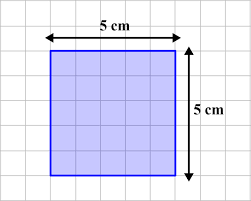To convert square miles to square centimeters, one essential parameter is needed and this parameter is Unit Value.

The formula for converting square miles to square centimeters:

1 Square Mile = 25899881103 Square Centimeters

Let’s solve an example;
Find the conversion of square miles to square centimeters when the unit value is 2.

1 Square Mile = 25899881103 Square Centimeters

Then,

2 Square Miles = (2 x 25899881103) Square Centimeters
2 Square Miles = 51799762206 Square Centimeters

Therefore, the square centimeter(s) is 51799762206.

## How to Convert Square Yards to Square Centimeters | Area Units

The image below represents square yards to square centimeters.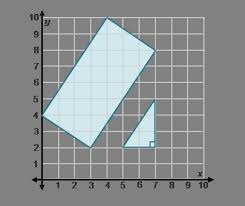To convert square yards to square centimeters, one essential parameter is needed and this parameter is Unit Value.

The formula for converting square yards to square centimeters:

1 Square Yard = 8361.274 Square Centimeters

Let’s solve an example;
Find the conversion of square yards to square centimeters when the unit value is 6.

1 Square Yard = 8361.274 Square Centimeters

Then,

6 Square Yards = (6 x 8361.274) Square Centimeters
6 Square Yards = 50167.644 Square Centimeters

Therefore, the square centimeter(s) is 50167.64

## How to Convert Square Feet to Square Centimeters | Area Units

The image below represents square feet to square centimeters.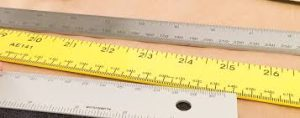To convert square feet to square centimeters, one essential parameter is needed and this parameter is Unit Value.

The formula for converting square feet to square centimeters:

1 Square Feet = 929.0304 Square Centimeters

Given an example;
Find the conversion of square feet to square centimeters when the unit value is 24.

1 Square Feet = 929.0304 Square Centimeters

Then,

24 Square Feet = (24 x 929.0304) Square Centimeters
24 Square Feet = 22296.7296 Square Centimeters

Therefore, the square centimeter(s) is 22296.72

## How to Convert Square Inches to Square Centimeters | Area Units

The image below represents square inches to square centimeters.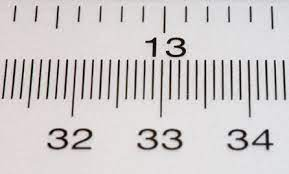To convert square inches to square centimeters, one essential parameter is needed and this parameter is Unit Value.

The formula for converting square inches to square centimeters:

1 Square Inch = 6.4516 Square Centimeters

Let’s solve an example;
Convert square inches to square centimeters when the unit value is 4.

1 Square Inch = 6.4516 Square Centimeters

Then,

4 Square Inches = (4 x 6.4516) Square Centimeters
4 Square Inches = 25.8064 Square Centimeters

Therefore, the square centimeter(s) is 25.80

## How to Convert Square Kilometers to Square centimeters | Area Units

The image below represents square kilometers to square centimeters.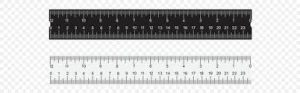The conversion of square kilometers to square centimeters requires one essential parameter and this parameter is Unit Value.

The formula for converting square kilometers to square centimeters:

1 Square Kilometer = 10000000000 Square Centimeters

Let’s solve an example;
Convert square kilometers to square centimeters when the unit value is 7.

1 Square Kilometer = 10000000000 Square Centimeters

Then,

7 Square Kilometers = (7 x 10000000000) Square Centimeters
7 Square Kilometers = 70000000000 Square Centimeters

Therefore, the square centimeter(s) is 70000000000.

## How to Convert Hectares to Square Centimeters | Area Units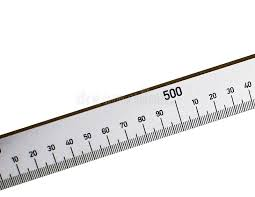The image above represents hectares to square centimeters.

The conversion of hectares to square centimeters requires one essential parameter and this parameter is Unit Value.

The formula for converting hectares to square centimeters:

1 Hectare = 100000000 Square Centimeters

Given an example;
Convert hectares to square centimeters when the unit value is 9.

1 Hectare = 100000000 Square Centimeters

Then,

9 Hectares = (9 x 100000000) Square Centimeters
9 Hectares = 900000000 Square Centimeters

Therefore, the square centimeter(s) is 900000000.

## How to Convert Square Meters to Square Centimeters | Area Units

The image below represents square meters to square centimeters.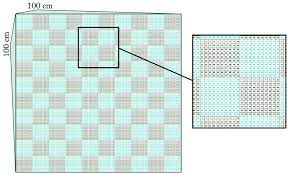The conversion of square meters to square centimeters requires one essential parameter and this parameter is Unit Value.

The formula for converting square meters to square centimeters:

1 Square Meter = 10000 Square Centimeters

Let’s solve an example;
Find the conversion of square meters to square centimeters when the unit value is 6.

1 Square Meter = 10000 Square Centimeters

Then,

6 Square Meters = (6 x 10000) Square Centimeters
6 Square Meters = 60000 Square Centimeters

Therefore, the square centimeter(s) is 60000.

## How to Convert Square Centimeters to Square Miles | Area Units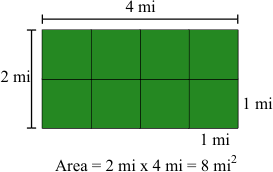The image above represents square centimeters to square miles.

The conversion of square centimeters to square miles requires one essential parameter and this parameter is Unit Value.

The formula for converting square centimeters to square miles:

1 Square Centimeter = 0.00000000003861 Square Miles

Given an example;
Convert square centimeters to square miles when the unit value is 10.

1 Square Centimeter = 0.00000000003861 Square Miles

Then,

1 Square Centimeters = 0.00000000003861 Square Miles
10 Square Centimeters = 3.8610000000000004e-10 Square Miles

Therefore, the square mile(s) is 3.861e-10.

## How to Convert Square Centimeters to Acres | Area Units

The image below represents square centimeters to acres.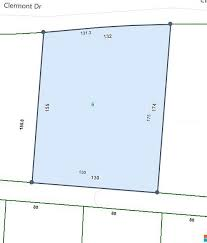The conversion of square centimeters to acres requires one essential parameter and this parameter is Unit Value.

The formula for converting square centimeters to acres:

1 Square Centimeter = 0.00000002471054 Acres

Given an example;
Convert square centimeters to acres when the unit value is 8.

1 Square Centimeter = 0.00000002471054 Acres

Then,

8 Square Centimeters = (8 x 0.00000002471054) Acres
8 Square Centimeters = 1.9768432e-7 Acres

Therefore, the acre(s) is 1.9768e-7.

## How to Convert Square Centimeters to Square Yards | Area Units

The image below represents square centimeters to square yards.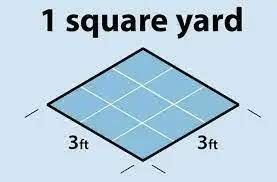The conversion of square centimeters to square yards requires one essential parameter and this parameter is Unit Value.

The formula for converting square centimeters to square yards:

1 Square Centimeter = 0.00012 Square Yards

Given an example;
Convert square centimeters to square yards when the unit value is 2.

1 Square Centimeter = 0.00012 Square Yards

Then,

2 Square Centimeters = (2 x 0.00012) Square Yards
2 Square Centimeters = 0.00024 Square Yards

Therefore, the square yard(s) is 0.00024.